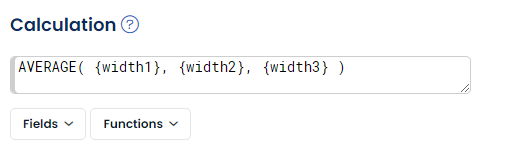# Function list

The versatile expression engine built into AlisQI enables users to create not only numeric calculations but also advanced business logic expressions. Combined with the workflows, this enables you to create business logic within AlisQI.

The expression engine is based on Twig, an open-source templating engine, and supports a rich syntax with many features, including functions, filters, tests, and operators.

On top of Twig's standard features, we added a number of extra functions to make your life just a bit easier. These functions can be found in the Function drop-down in AlisQI.# Numeric functions

• `ABS( value )` absolute value
• `AVERAGE( value, value, ..., value)` average of the non-empty values
• `MOVINGAVG( field, number )` moving average
• `DELTA( field )` difference compared to previous result
• `LOG( value, [base] )` log (base 10 by default)
• `POWER( base, power )` base raised to the power
• `MIN( value, value, ..., value )` minimum of non-empty values
• `MAX( value, value, ..., value)` maximum of non-empty values
• `STDEV( value, value, ..., value )` standard deviation of non-empty values
• `RANGE( value, value, ..., value )` range (max-min) for given fields or values
• `CEIL( value )` round fractions up (1.2 will be rounded to 2)
• `FLOOR( value )` round fractions down (1.9 will be rounded to 1)
• `COUNTRESULTS( {field1}.name, {field2}.name, {field3}.name )` count the number results with the same signature. The signature is defined by the three field parameters. Field 1 is mandatory, Fields 2 and 3 are optional.
Example: `COUNTRESULTS({batchcode})` will return a counter with the number of samples for this particular batchcode. This allows for first time right reporting.
• `COUNTRESULTS_BEFORE({datefield}.name, {field1}.name, {field2}.name, {field3}.name)` same as `COUNTRESULTS` but with a date and time reference, so that only results before the result's date will be counted.

`field` = field name of a numeric field (numeric or calculation)
`value` = field name of a numeric field or an expression

### Linear Regression

• `SLOPE( [x1,y1], [x2,y2], [x3,y3], ..., [x20,y20] )` Calculate the `a` in `y = ax+b`
• `INTERCEPT( [x1,y1], [x2,y2], [x3,y3], ..., [x20,y20] )` Calculate the `b` in `y = ax+b`
• `CORRELATION( [x1,y1], [x2,y2], [x3,y3], ..., [x20,y20] )` Calculate the correlation between all data points.

These linear regression functions all support up to 20 data points, defined as `[x,y]`.

A minimum set of 3 `x,y` pairs is required for each of the linear regression functions.

## Other numeric functions

The Twig engine that forms the core of our expression engine provides the following numeric functions:

# Date functions

## Date(time) difference

Subtracting two dates is possible using these two functions:

• `DATE_DIFF_MIN( dateField, dateField)` difference in minutes between date1 and date2
• `DATE_DIFF_DAY( dateField, dateField)` difference in days between date1 and date2
• `NOW()` return the current date + time, in the tenant's timezone.

## Compute a date(time)

To compute a date based on a reference date and offset, you can use:

• `DATE_ADD_DAY( dateField, offset)` offset can be a static integer value or reference to a field (incl. calculations)
• `DATE_ADD_MONTH( dateField, offset)` offset can be a static integer value or reference to a field (incl. calculations)

This will result in a new date (or datetime, depending on your field settings).

## Other date functions

The Twig engine that forms the core of our expression engine provides the following date functions:

The date and date_modify filters might come in handy when calculating date(time) values.
Example syntax to add 3 hours to the date field's value and convert it to yyyy-mm-dd hh:mm:ss:
```{date}|date_modify("+3 hours")|date("Y-m-d H:i:s")
```

# Text functions

Text calculation fields can use the following function:
• `CONCAT ( value, value, value, ...)`  This function glues all values together. Values can either be field names or static text strings.
`CONCAT({code}, ' - ', {name})` with code = 123 and name = ABC will result in "123 - ABC"

A short hand notation for concatenating values is the `~` operator.
`{field1}~{field2}` will glue the values for fields 1 and 2 together.

## Regular expressions

Regular expressions provide a powerful and flexible way to search for and manipulate text patterns. Our regex filters allows for advanced pattern matching and extraction, making it a valuable tool for text processing tasks. The syntax is based on the Perl Compatible Regular Expressions (PCRE) syntax.

• preg_quote
• preg_match
• preg_get
• preg_get_all
• preg_grep
• preg_replace
• preg_filter
• preg_split

## Nesting in expressions

Value parameters can either be field names or expressions. This allows for function nesting, where the output of a function is used as input for another function.

The `DELTA` and `MOVINGAVG` functions only accept a field name as parameter and no expressions. This is due to the fact that these functions evaluate multiple results (rows in the results overview).

# Filters

Variables can be modified by filters. Filters are separated from the variable by a pipe symbol ( `|`). Multiple filters can be chained. The output of one filter is applied to the next.

The 'upper' filter would convert 'diana' into 'DIANA'

```{name_}|upper
```